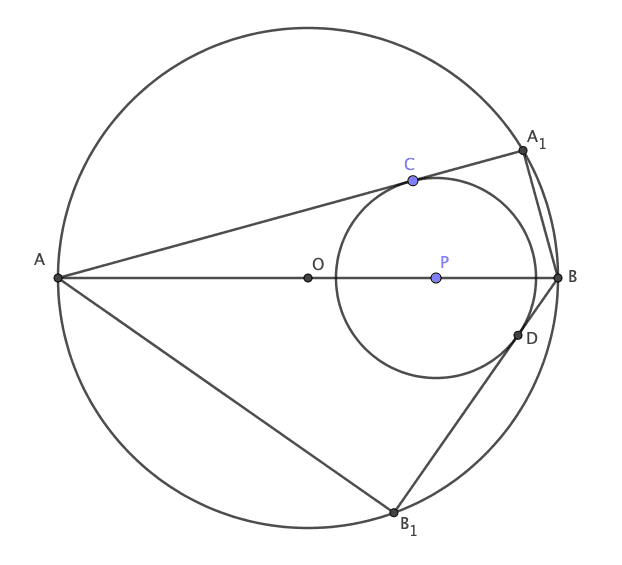How Cheenta works to ensure student success?
Explore the Back-Story

# Circle in Circle - PRMO 2017 | Problem 27Let $\Omega_1$ be a circle with center O and let AB be a diameter of $\Omega_1$. Let P be a point on the segment OB different from O. Suppose another circle $\Omega_2$ with center P lies in the interior of $\Omega_1$. Tangents are drawn from A and B to the circle $\Omega_2$ intersecting $\Omega_1$ again at $A_1$ and $B_1$ respectively such that $A_1$ and $B_1$ are on the opposite sides of AB. Given that $A_1B = 5, AB_1 = 15$ and $OP = 10$, find the radius of $\Omega_1$.

Do you really need a hint? Try it first!

Hint 1

Draw a diagram carefully.Hint 2

Suppose the point of tangencies are at C and D. Join PC and PD.

Can you find two pairs of similar triangles?Hint 3

$\Delta APC \sim \Delta AA_1B$

Why?

Notice that AC is perpendicular to $AA_1$ as the radius is perpendicular to the tangent.

Also $\angle A$ is common to both triangles. Hence the two triangles are similar (equiangular implies similar).

Similarly $\Delta BPD \sim \Delta BAB_1$.

Use the ratio of sides to find OA.

Hint 4

Suppose OA = R (radius of the big circle).

OC  = r (radius of the small circle).

We already know OP = 10, $A_1 B = 5, AB_1 = 15$Since $\Delta AA_1B$ and $ACP$ are similar we have $\frac{AP}{AB} = \frac{PC}{A_1B}$. This implies  $\frac{R+10}{2R} = \frac{r}{5}$ (1)

Similarlly since $\Delta BPD$ and $BAB_1$ are similar we have $\frac{BP}{BA} = \frac{PD}{AB_1}$. This implies  $\frac{R-10}{2R} = \frac{r}{15}$ (2)

Multiply the reciprocal of (2) with (1) to get R = 20.

# Connected Program at Cheenta

Math Olympiad is the greatest and most challenging academic contest for school students. Brilliant school students from over 100 countries participate in it every year.

Cheenta works with small groups of gifted students through an intense training program. It is a deeply personalized journey toward intellectual prowess and technical sophistication.

Let $\Omega_1$ be a circle with center O and let AB be a diameter of $\Omega_1$. Let P be a point on the segment OB different from O. Suppose another circle $\Omega_2$ with center P lies in the interior of $\Omega_1$. Tangents are drawn from A and B to the circle $\Omega_2$ intersecting $\Omega_1$ again at $A_1$ and $B_1$ respectively such that $A_1$ and $B_1$ are on the opposite sides of AB. Given that $A_1B = 5, AB_1 = 15$ and $OP = 10$, find the radius of $\Omega_1$.

Do you really need a hint? Try it first!

Hint 1

Draw a diagram carefully.Hint 2

Suppose the point of tangencies are at C and D. Join PC and PD.

Can you find two pairs of similar triangles?Hint 3

$\Delta APC \sim \Delta AA_1B$

Why?

Notice that AC is perpendicular to $AA_1$ as the radius is perpendicular to the tangent.

Also $\angle A$ is common to both triangles. Hence the two triangles are similar (equiangular implies similar).

Similarly $\Delta BPD \sim \Delta BAB_1$.

Use the ratio of sides to find OA.

Hint 4

Suppose OA = R (radius of the big circle).

OC  = r (radius of the small circle).

We already know OP = 10, $A_1 B = 5, AB_1 = 15$Since $\Delta AA_1B$ and $ACP$ are similar we have $\frac{AP}{AB} = \frac{PC}{A_1B}$. This implies  $\frac{R+10}{2R} = \frac{r}{5}$ (1)

Similarlly since $\Delta BPD$ and $BAB_1$ are similar we have $\frac{BP}{BA} = \frac{PD}{AB_1}$. This implies  $\frac{R-10}{2R} = \frac{r}{15}$ (2)

Multiply the reciprocal of (2) with (1) to get R = 20.

# Connected Program at Cheenta

Math Olympiad is the greatest and most challenging academic contest for school students. Brilliant school students from over 100 countries participate in it every year.

Cheenta works with small groups of gifted students through an intense training program. It is a deeply personalized journey toward intellectual prowess and technical sophistication.

This site uses Akismet to reduce spam. Learn how your comment data is processed.

### 7 comments on “Circle in Circle - PRMO 2017 | Problem 27”

1.Asmit Paria says:

radius of omega1 is 30 units. The problem is good but send me some elementary and difficult number theory, algebra,combinatorics problems and some good study material lecture vidoes or books for PRE- RMO, RMO, INMO and also for ISI and CMI PREPARATION. Send me as early as possible.

1.Ashani Dasgupta says:

Our computations show it is 20. We double checked with HBCSE. Can you check your calculations? If after reviewing if you think your calculations are correct, then do share it with us.

2.Asmit Paria says:

send more varieties of problems.

1.Ashani Dasgupta says:

Sure.

3.DEB JYOTI MITRA says:

Nicely Illustrated & Hinted in stages.I like it,It is valuable for all concerned with it.I wish a grand success for thiss endeavour.

1.Ashani Dasgupta says:

Thank you! Keep solving.

4.Arghaya Maulik says:

Triangle APC and Triangle ABA1 are similar. If r is the radius of smaller circle and R is the radius of bigger circle then,we obtain the equation:
(r/5)=(R+10)/2R.
Similarly, Triangle BPD and Triangle BAB1 are also similar. Hence we get another equation as:
(r/15)=(R-10)/2R.
Hence on solving for R we get 2R=10+30, or, R=20... That's the answer. It's easy but a good problem. THANKYOU. I would like to request you to provide few problems for CMI and IOMA preparation as ISI is knocking the door!

### Knowledge Partner